Homework Explained - Math Practice 101Dear guest, you are not a registered member. As a guest, you only have read-only access to our books, tests and other practice materials.

As a registered member you can:

Registration is free and doesn't require any type of payment information. Click here to Register.
Go to page:
Chapter 9:Area;Lesson 1: Area of Parallelograms

Find the area of each parallelogram.

• Question 13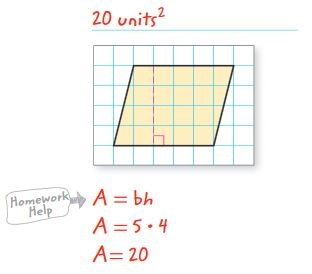• Question 14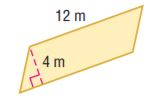•  $$\text{ m}^2.$$
• Question 15

base, 12 inches;
height, 15 inches

•  $$A =$$ $$\text{ in}^2.$$
• Question 16

Find the height of a parallelogram with base 6.75 meters and an area of 218.7 square meters.

•  h = m
• Question 17

Find the area of a parallelogram with base 15 yards and height $$21\frac{2}{3}$$ yards.

•  $$A =$$ $$\text{ yd}^2.$$
• Question 18

What is the area of the region shown on  the map?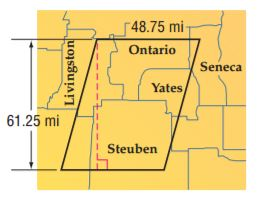•  $$A =$$ $$\text{ mi}^2.$$
• Question 19

What is the height of the parallelogram shaped pattern block shown below?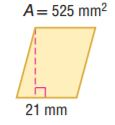•  $$h =$$ $$\text{ mm}$$

Draw and label each figure. Then find the area.

• Question 20

a parallelogram with an equal base and height  and an area greater than 64 square meters

• Question 21

a parallelogram with a base four times the height and an area less than 200 square feet

Identify Structure Find the area of the shaded region in each figure.

• Question 22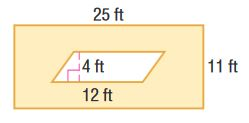•  $$\text{ ft}^2$$
• Question 23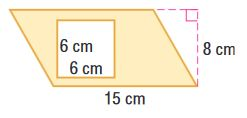•  $$\text{ cm}^2.$$

Yes, email page to my online tutor. (if you didn't add a tutor yet, you can add one here)